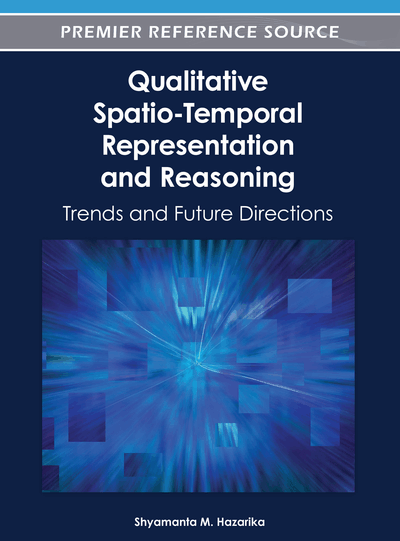# Explanation Generation over Temporal Interval Algebra

Debasis Mitra (Florida Institute of Technology, USA) and Florent Launay (Florida Institute of Technology, USA)
DOI: 10.4018/978-1-61692-868-1.ch008

## Abstract

Temporal interval algebra has generated strong interest for both theoretical and practical reasons. All its Maximal Tractable Subalgebras (MTS) have been identified. Now is the time to make the transition toward their practical applications. In this chapter, the authors have proposed a formalism on how to classify an input temporal network in one of these MTSs or decide its intractability. They have also proposed a linear algorithm for checking consistency when the input belongs to one of the seventeen MTSs, and for finding the constraints responsible for inconsistency in case the network is unsatisfiable.
Chapter Preview
Top

## Background On Temporal Reasoning

Qualitative reasoning with intervals involves thirteen atomic relations, B: {before(p), after(p-1), meets(m), met-by(m-1), overlaps(o), overlapped-by(o-1), starts(s), started-by(s-1), during(d), contains(d-1), finishes(f), finished-by (f -1), equal(eq)}, between any pair of intervals (Allen, 1983). The corresponding relational algebra is comprised of the power set P(B), the power set of B, which is closed under the traditional reasoning operators like composition, converse, set union, and set intersection.

Definition 1: A Qualitative Temporal Constraint Network (QTCN) is a graph G=(V, E), where each node denotes an interval, and each directed labeled edge (v1, v2, R) ∈ E represents disjunctive constraint R between v1 and v2, where RP(B). Two special relations are tautology (disjunction of all thirteen atomic relations or no constraint), and Ø (empty relation leading to inconsistency). Reasoning may be restricted to a subset Θ, where R ∈ Θ ⊆ P(B), in case Θ is closed under composition, converse and intersection, thus, forming a Θ-subalgebra.

## Complete Chapter List

Search this Book:
Reset